Yoshihara, H. (2013). "Mode II critical stress intensity factor of medium-density fiberboard measured by asymmetric four-point bending tests and analyses of kink crack formation," BioRes. 8(2), 1771-1789.

#### Abstract

Using medium-density fiberboard specimens, asymmetric four-point bending tests were conducted to obtain the Mode II critical stress intensity factor for this in-plane system. Because the medium-density fiberboard is in-plane quasi-isotropic about its board plane, the crack propagates obliquely with respect to the initial crack direction under the asymmetric four-point loading condition. A finite element analysis is required to obtain the Mode II stress intensity factor. The analysis herein was conducted to take into account the kink crack formation. In addition, a three-point bend end-notched flexure test was also conducted, and the results obtained by the experiments and numerical calculations were compared. When the initial crack’s length-to-specimen’s depth ratio ranged from 0.85 to 0.95 and when the additional crack length was taken into account, the Mode II critical stress intensity factor KIIc was appropriately obtained by the asymmetric four-point bending test.

Mode II Critical Stress Intensity Factor of Medium-Density Fiberboard Measured by Asymmetric Four-Point Bending Tests and Analyses of Kink Crack Formation

Hiroshi Yoshihara

Using medium-density fiberboard specimens, asymmetric four-point bending tests were conducted to obtain the Mode II critical stress intensity factor for this in-plane system. Because the medium-density fiberboard is in-plane quasi-isotropic about its board plane, the crack propagates obliquely with respect to the initial crack direction under the asymmetric four-point loading condition. A finite element analysis is required to obtain the Mode II stress intensity factor. The analysis herein was conducted to take into account the kink crack formation. In addition, a three-point bend end-notched flexure test was also conducted, and the results obtained by the experiments and numerical calculations were compared. When the initial crack’s length-to-specimen’s depth ratio ranged from 0.85 to 0.95 and when the additional crack length was taken into account, the Mode II critical stress intensity factor KIIc was appropriately obtained by the asymmetric four-point bending test.

Keywords: Medium-density fiberboard; Critical stress intensity factor; Asymmetric four-point bending test; Finite element analysis; Virtual crack closure technique; Kink crack

Contact information: Faculty of Science and Engineering, Shimane University, Nishikawazu-cho 1060, Matsue Shimane 690-8504, Japan; yosihara@riko.shimane-u.ac.jp

INTRODUCTION

Medium-density fiberboard (MDF) is one of the principal wood products used for various construction projects; therefore, a better understanding of its mechanical properties, including its fracture mechanical properties, is required.

The fracture mechanics of MDF has been considered in several previous studies (Niemz et al. 1997, 1999; Olbert and Schenck 1997; Suzuki and Matsushita 1998; Olbert 1999; Nairn 2009; Matsumoto and Nairn 2009; Yoshihara 2010). Nevertheless, most of these studies have been limited to the study of Mode I (crack opening mode) fracture mechanics. In contrast, there have been only a few studies that investigated Mode II (in-plane shear mode) fracture mechanics. An exception is a study by the author of the present study, in which the three-point bend end-notched flexure (3ENF) test for measuring the relationship between the propagation fracture toughness and the crack length (resistance curve; i.e., R-curve) was conducted (Yoshihara 2010).

The 3ENF test is advantageous for measuring the Mode II fracture mechanics parameters because the fracture mechanics parameters can be obtained from beam theory, which is based on energy considerations and is mathematically well-defined (Adams et al. 2003). As shown in the previous study by the present author (Yoshihara 2010), it was difficult to conduct 3ENF for MDF without cutting grooves on the side surfaces to enhance crack propagation while preventing bending failure. Nevertheless, tests using a side-grooved specimen have a drawback – the crack path deviates from the general direction along which the crack should propagate.

In general, cracks contained in fibrous materials, such as wood, propagate along the fiber direction; therefore, when the initial crack direction coincides with the fiber direction, it is rather easy to analyze the fracture mechanics because of the coincidence of these directions. In an in-plane quasi-isotropic system such as the board plane of MDF, however, the cracks often propagate obliquely in the board plane under pure Mode II loading conditions. This oblique propagation phenomenon is described below in detail. Oblique propagation is related to kink formation; therefore, to analyze the Mode II fracture mechanics of MDF, kink crack formation should be taken into account. The asymmetric four-point bending (AFPB) test of a single-edge-notched (SEN) specimen, the details of which are described below, can be conducted without cutting grooves on the side surfaces, enabling the analysis of Mode II fracture mechanics while considering the kink crack formation.

In the present study, AFPB tests were performed on MDF using SEN specimens with various crack lengths. The crack geometry factor, which is required to calculate the Mode II critical stress intensity factor KIIc, was obtained using finite element analyses (FEAs), which included consideration of kink crack formation. In addition, 3ENF tests were independently conducted. The Mode II fracture toughness, GIIc, obtained by the 3ENF test was transformed into KIIc. The validity of the AFPB test was examined by comparing the results from experiments and numerical calculations.

Kink Crack Formation under the Asymmetric Four-Point Bending Test of Single-Edge-Notched Specimens

Figure 1 presents a schematic diagram of the AFPB test of a SEN specimen. This test has been originally developed for examining the fracture behaviors of isotropic material under various mixed Mode I/II conditions (Suresh et al. 1990; Fett et al. 1995; Li and Sakai 1996; He and Hutchinson 2000; Choi et al. 2003, 2005). When the location of the crack is varied with respect to the center plane of the specimen, the mixed-mode ratio can be controlled. As in this research, however, the pure Mode II condition is expected when the crack is located at the center plane.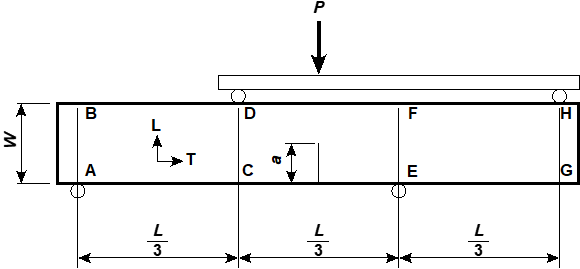Fig. 1. A diagram of the asymmetric four-point bending (AFPB) test of a single-edge-notched bending (SENB) specimen

The specimen was eccentrically supported at two trisected points, and loads were applied at the remaining two points. The crack length is defined as a, and the distance between the left loading point and the right supporting point is defined as L. The directions parallel and perpendicular to the crack surface are defined as x and y, respectively. This fracture test has been previously conducted to measure Mode II fracture mechanics of ceramics (Suresh et al. 1990; Fett et al. 1995; Li and Sakai 1996; He and Hutchinson 2000; Choi et al. 2003, 2005), and later it was applied to solid wood (Yoshihara 2008, 2012) and composite materials with a glue line at the center (Krishnan and Xu 2010).

The Mode II stress intensity factor KII is derived using the following equation (Suresh et al. 1990; Fett et al. 1995; Li and Sakai 1996; He and Hutchinson 2000; Choi et al. 2003, 2005),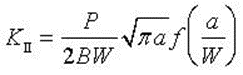(1)

where B and W are the beam width and depth, respectively, and f(a/W) is the crack geometry factor. The term f(a/W) is usually determined by FEAs. The details of the calculation of this last term are described below.

In an orthotropic material, such as wood, cracks usually propagate along the fiber direction. When Mode II mechanics are the dominant condition, such as in isotropic materials, the crack does not propagate straight from the crack tip. Rather, it propagates obliquely with respect to the initial direction (Suresh et al. 1990; Fett et al. 1995; Li and Sakai 1996; He and Hutchinson 2000; Choi et al.2003, 2005). Several previous studies suggested that the anisotropy of MDF sheets can arise because of the directionality that is induced during the manufacturing process (Kitahara 1963; Kazemi Najafi et al. 2007). To confirm the quasi-isotropy of the MDF sheet, off-axis specimens were therefore cut from the sheet, and the Young’s modulus and shear modulus corresponding to the off-axis angle were measured by a flexural vibration test, whereas the Poisson’s ratio was measured by a tension test. Figure 2 shows the elastic constants corresponding to the off-axis angle. From this figure, the elastic constants can be regarded as being independent of the off-axis angle.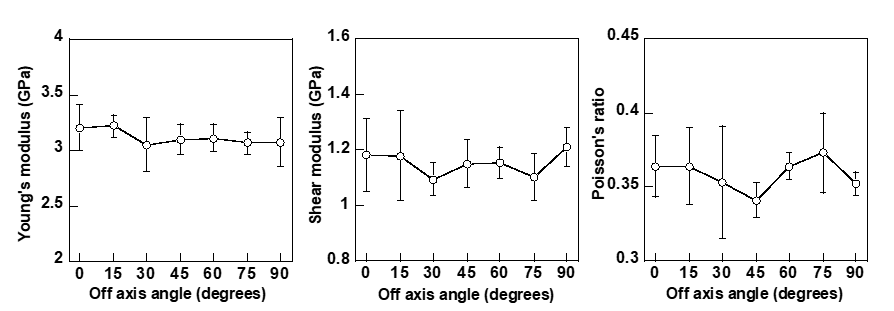Fig. 2. Young’s modulus, shear modulus, and Poisson’s ratio corresponding to the off-axis angle of the MDF sheet. Unfilled circles and vertical bars represent the average and standard deviations, respectively.

Additionally, the GIc values obtained from this MDF panel were 1.60 0.27 and 1.78 0.34 J/m2 for the TL and LT systems, respectively, whereas the GIIc values of TL and LT systems were 2.58 0.25 and 2.41 0.30 J/m2, respectively (Yoshihara 2010a). Therefore, there were no significant differences between the fracture toughness values obtained from the different systems. From these results, the MDF sheet investigated in this study can be regarded to be in-plane quasi-isotropic about its board plane.

Several studies have been conducted on kink crack formation in isotropic bodies (Nuismer 1974; Bilby and Cardew 1975; Hayashi and Nemat-Nasser 1981; Kageyama and Okamura 1982). Among them, Kageyama and Okamura (1982) proposed a criterion for kink crack formation under a generalized Mode I and Mode II combined state. When the directions parallel and perpendicular to the kink crack surface are defined as X and Y, respectively, and the angle between the x– and X-directions is defined as  as shown in Fig. 3d, the Kageyama and Okamura’s criterion is derived as follows,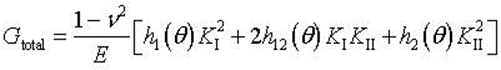(2)

where Gtotal is the total strain energy release rate, KI and KII are the Mode I and Mode II stress intensity factors before the occurrence of kink crack, respectively, which are each defined according to the initial crack direction (xy coordinate system). The parameter E is Young’s modulus under plane strain conditions. The parameters h1(), h12(), and h2() are represented as follows,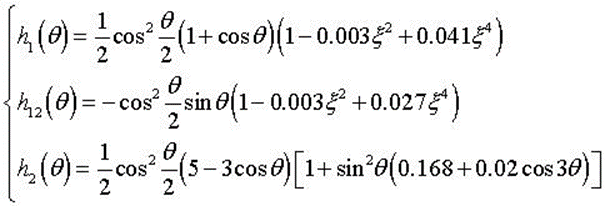(3)

where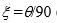(degrees). The value of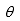is defined as the angle that allows the maximum value of Gtotal. Under the pure Mode II conditions, KI = 0, so Eq. (2) can be simplified as follows: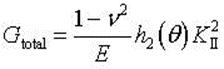(4)

Figure 4 shows the relationship between the parameter h2) and. When= 76°, the value of h2) is at its maximum and derived as 1.52. Thus, Eq. (4) is: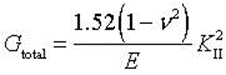(5)

As shown in Figs. 3(a)-(c), the crack propagated in a curve when it approached the loading point. At the initiation of the crack propagation, the experimentally-obtained value ofwas 75.6 ± 5.8°. In addition, the value of h2) did not vary significantly from the= 76°. From these issues, it is concluded that the crack propagated under a rather pure Mode II condition and that Eq. (5) is effective for analyzing the formation of kink cracks in the AFPB test.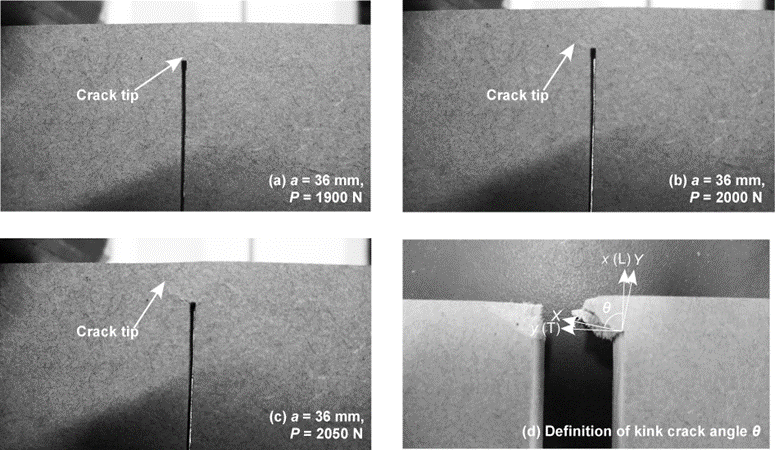Fig. 3. Kink crack formation under the asymmetric four-point bending (AFPB) test of a single-edge-notched bending (SENB) specimen of medium-density fiberboard (MDF). The angle between the xand X axes,, is approximately 76 at the initiation of crack propagation. L and T represent the length and the width (transverse direction) of the MDF sheet, respectively.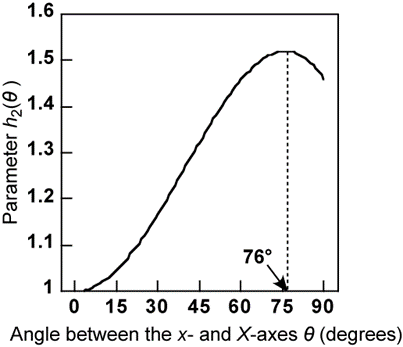Fig. 4. The relationship between the parameter h2) and the angle between the x– and X-axes,

Finite Element Analyses (FEA)

Two-dimensional FEAs were conducted using the virtual crack closure technique (VCCT). The FE program utilized was ANSYS 12. Figure 5 shows the FE model of a representative specimen. The horizontal length of the model was 260 mm, and the model depth W and model width B were 40 mm and 15 mm, respectively. The model consisted of four-noded plane elements similar to previous studies by the author (Yoshihara 2010b, c, 2012; Yoshihara and Satoh 2009; Yoshihara and Usuki 2011). Although the finite element mesh was confirmed to be fine enough, further research should be undertaken on the model containing the special elements to simulate the crack tip singularity (Xie et al. 2004). The analysis was conducted under plane stress conditions.

Similar to the actual AFPB test, the vertical and horizontal directions of the model were defined as the x– and y-directions so that the direction of the initial crack coincided with the x-axis. The mesh size of the model was refined near the crack tip. As described above, a kink crack was always induced in the AFPB test of MDF, as shown in Fig. 3. FE models for the unkinked and kinked states were prepared based on the following “two-step-analysis approach” (Xie et al. 2004). Figures 5(b) and (d) show the details of the FE models in the unkinked state, whereas Figs. 5(c) and (e) show those in the kinked state. For both models, the dimensions of the elements around the crack tip were 0.1 0.1 mm2. As described above, the crack under pure Mode II loading conditions initially propagated in the direction inclined at 76° with respect to the initial crack direction; therefore, the geometrical axis of the elements around the crack tip was inclined at 76° with respect to the initial crack direction in the kinked state as shown in Figs. 5(b) and (d). Similar to the actual AFPB test, the directions parallel and perpendicular to the kink crack direction were also defined as the X and Y directions, respectively. The initial crack length, a, varied from 28 to 38 mm at intervals of 2 mm; therefore, the crack length-to-specimen’s depth ratio, a/W, varied from 0.7 to 0.95 in intervals of 0.05. The Young’s modulus, E, and Poisson’s ratio,  used in the present calculations were 3.2 GPa and 0.36, respectively; thus, the shear modulus, G (= E/(2 + 2)), used was 1.18 GPa. As described above, the Young’s modulus and Poisson’s ratio values were determined from the flexural vibration and tension tests, respectively. The model was supported in the vertical direction at y = 90 and 250 mm at the bottom surface, and a vertical displacement ux of 1 mm was applied to the nodes at y = 10 and 170 mm downward at the top surface. Hence, the distance between the left supporting point and the right loading point L was 240 mm, giving L/W = 6 in this simulation.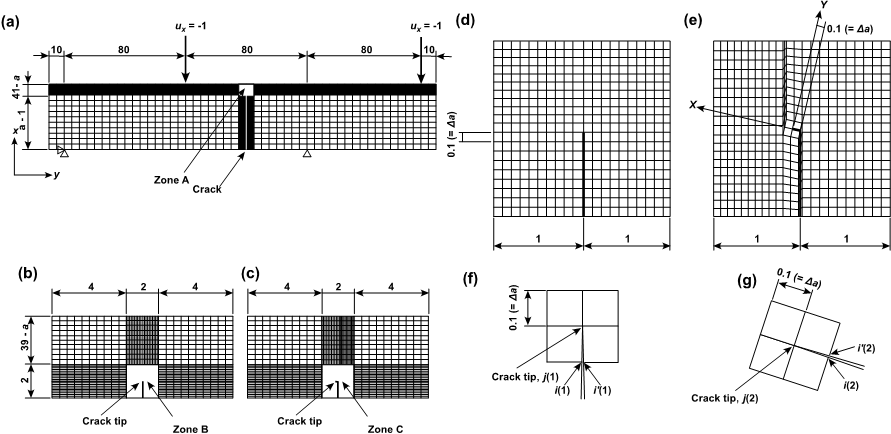Fig. 5. The finite element model used for the SEN-AFPB test analysis: (a) the overall mesh, (b) the detail of zone A in (a) at the unkinked state, (c) the detail of zone A in (a) at the kinked state, (d) the detail of zone B in (b), (e) the detail of zone C in (c), (f) the detail around the crack tip at the unkinked state, and (g) the detail around the crack tip at the kinked state. The angle between the x- and X-axes is 76.

The Mode I and Mode II strain energy release rates in the unkinked state, GI(1) and GII(1), respectively, were calculated using the following equation (Rybicki and Kanninen 1977),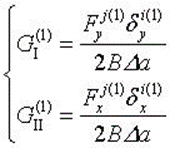(6)

where Fxj(1) and Fyj(1) are the nodal forces at the crack tip node j(1), which is shown as Fig. 5(f), in the x and y directions, respectively. The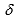xj(1) andyj(1) variables are the relative displacements between the nodes i(1) and i’(1), respectively, which are located at a distance of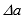mm behind the crack tip as shown in Fig. 5(f). These displacements are calculated from the state before the kink crack propagation using the model represented by Figs. 5(a), (b), and (d). The value of Gtotal(1) is defined as follows: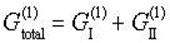(7)

From the values of GI(1)/Gtotal(1) and GII(1)/Gtotal(1), the mixed-mode ratio before the kink crack formation was evaluated.

There is a concern that the strain energy release rate produced by the kink crack formation cannot be evaluated appropriately using Eq. (6), which is based on the hypothesis that the crack propagates collinearly from the initial crack tip; therefore, the post crack formation Mode I and Mode II strain energy release rate components, GI(2) and GII(2), were calculated based on the two-step-analysis approach (Xie et al. 2004). From the results of unkinked and kinked models, the Mode I and Mode II strain energy release rates in the kinked state, GI(2) and GII(2), were obtained as follows (Xie et al.2004),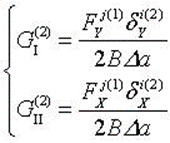(8)

where FXj(1) and FYj(1) are the nodal forces at the crack tip node j(1) in the X and Y directions, respectively, which were calculated from the unkinked state using the model represented by Figs. 4(a), (b), and (d). In contrast,xj(2) andyj(2) are the relative displacements between nodes i(2) and i’(2) in the X and Y directions (Fig. 5(g)), respectively, which were calculated from the kinked state using the model represented in Figs. 5(a), (c), and (e). The total energy release rate for closing the kink crack Gtotal(2) is thus derived as follows: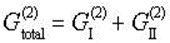(9)

The value of Gtotal(2) can be regarded as Gtotal in Eq. (5); therefore, the crack geometry factor f(a/W) is derived from Eqs. (1), (2), (5), and (9), eliminating KII as follows: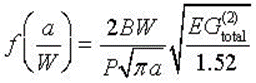(10)

By substituting the total load, P, applied to the FE model and the total energy release rate Gtotal(2), which was calculated by inserting the VCCT into this equation, the value of f(a/W) corresponding to the initial crack length-to-specimen’s depth ratio a/W was obtained. In addition, the mixed-mode ratio after the kink crack formation was evaluated using the values of GI(2)/ Gtotal(2) and GII(2)/ Gtotal(2).

Experimental

Materials

An MDF sheet sized to 1820 x 910 x 15 mm3 (length x width x thickness) was used to obtain the test specimens for this study. The board was fabricated in a board mill using softwood with a typical fiber length of 2 to 4 mm and urea-formaldehyde (UF) resin. The resin content of the MDF sheet was 7%. It had a density of 0.58 0.01 g/cm3 and was stored in a room kept at 20ºC and 65% relative humidity before testing. The moisture content in the air-dry condition was 10%. All of the specimens were cut from this board.

Similar to the definitions in previous studies (Nairn 2009; Matsumoto and Nairn 2009), the directions along the length, width, and thickness of the board were defined as the L, T, and Z directions, respectively, and an in-plane crack system (TL-system) was examined. The first and second letters, T and L, represent the direction normal to the crack surface and the direction along the crack length, respectively.

Asymmetric Four-Point Bending Test of a Single-Edge-Notched Specimen

In the AFPB test of the SEN specimen, two types of specimens were used to examine the scale effect on the value of KIIc. The larger specimens had dimensions of 40 260 15 mm3 in the x (L), y (T), and zdirections of the MDF sheet, respectively, while these dimensions were 20 130 15 mm3 for the smaller specimens; therefore, the specimen depth, W, was 40 mm for the larger specimens and 20 mm for the smaller specimens. A crack was produced in the mid-span. The crack was first cut with a band saw (thickness of 1 mm) and then extended 1 mm ahead of the crack tip using a razor blade. The extension of the crack was performed under a magnifying glass. The crack length a varied from 28 to 38 mm at an interval of 2 mm in the larger specimens and from 14 to 19 mm at an interval of 1 mm in the smaller specimens. Thus, the value of a/W varied from 0.7 to 0.95 at an interval of 0.05. These conditions were similar to those used in the FEA. In preliminary tests, failure by bending was often induced at points C or F in Fig. 1 before crack propagation occurred when the value of a/W was less than 0.7. The range of a/W was therefore determined as described above to induce crack propagation before the occurrence of bending failure.

In the 3ENF tests, the detail of which is described in a previous report (Yoshihara 2010a), grooves were cut along the neutral axis of both side surfaces to enhance the crack propagation while preventing the specimen from bending failure. In the MDF sheet, however, there is a density profile in the thickness direction, and the density at the region close to its surface is usually larger than that close to the mid-thickness (Bodig and Jayne 1982; Schulte and Früwald 1996; Dunky and Niemz 2002). When the groove is inserted on the surface of the specimen, the portion with a large density is cut away, so the KIIc value of the side-grooved specimen may be different from that of the groove-free specimen. To examine the influence of the grooves in the AFPB test, side-grooved specimens were prepared using specimens with dimensions similar to those described above. Grooves with a width of 1.5 mm and a depth of 6 mm were cut along the depth direction at the mid-length in both side surfaces of the specimen, so the remaining ligament thickness was 3 mm. The groove width and thickness were similar to those of the 3ENF specimen, the detail of which is described below. Consequently, the following four types of specimens were prepared: larger and groove-free, smaller and groove-free, larger and grooved, and smaller and grooved.

As shown in Fig. 1, the specimens were asymmetrically supported and loaded. The total span length L, which corresponds to the distance between the left supporting point and the right loading point, was 240 and 120 mm in specimens A and B, respectively. These values give an L/W ratio of 6. A steel platen was placed between the specimen and the support to reduce indentation at the supporting point. The platen could rotate around the supporting point without any indentations and did not interrupt deformation of the specimen. Platens with lengths equal to 30 mm and 15 mm were used for testing the larger and smaller specimens, respectively. Table 1 shows the crosshead speed corresponding to the testing conditions. The load P was applied until the specimen split into two pieces or the load decreased to the half of its maximum. The total testing time was approximately 5 min each. Five specimens were used for each a/W ratio, so the total specimen numbers were 120.

Table 1. The Crosshead Speed Corresponding to the Testing Conditions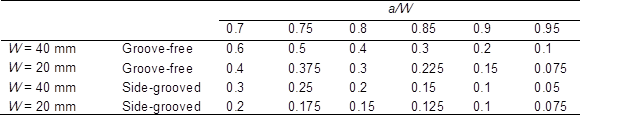*unit: mm/min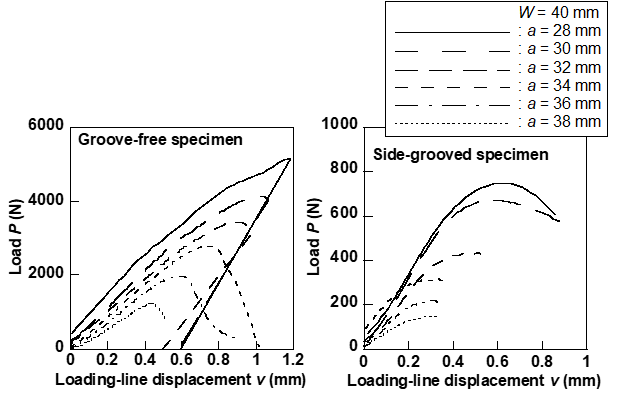Fig. 6. Typical examples of the load/loading-line deflection relationship obtained in the AFPB test of SEN specimen of MDF

Three-Point Bend End-Notched Flexure Tests

The value of GIIc was measured using the 3ENF test independently of the AFPB test, and it was transformed into KIIc. The values of KIIc obtained by the 3ENF test were compared with those obtained by the AFPB test. As described above, it was difficult to conduct the 3ENF of MDF without cutting grooves on the side surfaces to enhance crack propagation while preventing the specimen from bending failure (Yoshihara 2010a); therefore, grooves with a width of 1.5 mm and a depth of 6 mm were cut along the neutral axis on both side surfaces. The details of the 3ENF test using this specimen was described in a previous report (Yoshihara 2010a). The critical load for crack propagation was defined as the load at which the onset of nonlinearity was observed in the 3ENF test. The initiation fracture toughness, GIIc, was obtained from the critical load. Because it appears that an almost pure Mode II condition is found in the 3ENF test, the value of KIIc was obtained by substituting GIIc into Gtotal in Eq. (5). The Young’s modulus in Eq. (5) was determined from the load-longitudinal strain relationship in the 3ENF test.

In the 3ENF test, the relationship between the load and the loading-line deflection deviated from that predicted by Timoshenko’s beam theory, in which the deflection caused by the shearing force and the bending moment is taken into account. Similar to the analysis conducted in solid wood (Yoshihara and Satoh 1999), the influence fracture process zone (FPZ), the deformation caused by crack tip rotation, and the shear deformation of the beam ahead of the crack tip was taken into account as the additional crack length.

Results and discussion

Finite Element Analyses (FEA)

Figure 7 shows comparisons of the mixed-mode ratios before and after the kink crack formation obtained by the FEA, which were calculated from Eqs. (6) and (8), respectively. At the state before the kink crack formation, the Mode II component exceeds 99% of the total strain energy release rate; therefore, an almost pure Mode II condition can be found in the AFPB test of MDF. In the state after the kink crack formation, however, the Mode II component markedly decreases, and the Mode I component exceeds 90% of the total strain energy release rate. This issue indicates that the crack propagates under a Mode I dominant condition once the kink crack is initiated. In a previous work, the 3ENF test of MDF was conducted using a side-grooved specimen, and it was difficult to obtain the Mode II R-curve (Yoshihara 2010). The FEA results indicate that it may be essentially impossible to obtain the R-curve of MDF under a pure Mode II condition.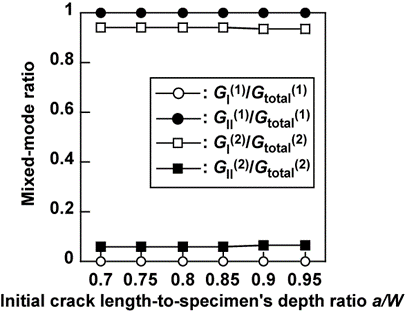Fig. 7. The mixed-mode ratio before and after the kink crack formation obtained by the FEA

In studies by Fett et al. (1995) and He and Hutchinson (2000), the relationship between f(a/W) and a/Wwas derived as follows:

Fett et al.: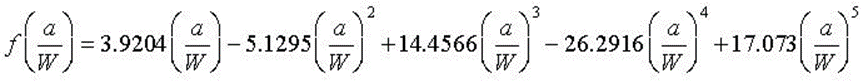(11)

He and Hutchinson: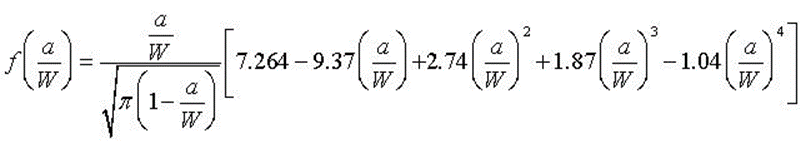(12)

In the present study, the relationship between f(a/W) and a/W obtained by the FEA was regressed into a 3rd-order polynomial equation because it could be approximated better with a linear or 4th order polynomial functions (Yoshihara 2012). The obtained equation is: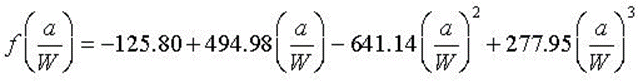(13)

Figure 8 shows the relationships between the crack geometry factor f(a/W) and the crack length-to-specimen’s depth ratio a/W obtained by the FEAs and compares it with the relationships derived in the previous studies. As described earlier, the kink crack formation was not taken into account in the analyses of the quoted authors. The FEA results that consider kink crack formation coincide well with the analyses of the quoted authors as well as those conducted in this study, except for the f(a/W) value derived by Eq. (11) at a/W of 0.95. Although the equations proposed by the quoted authors derive the proper relationship between f(a/W) and a/W, Eq. (13) is used for the analysis of fracture mechanics of MDF obtained by the actual AFPB test because it is appropriate to use results obtained while considering the kink crack formation. The mesh used in this study was confirmed to be fine enough that the crack geometry factor was not dependent on the mesh size.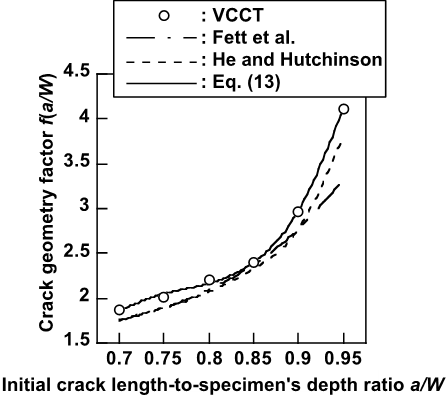Fig. 8. The relationships between the crack geometry factor f(a/W) and the initial crack length-to specimen’s depth ratio a/W obtained by the virtual crack closure technique (VCCT) and the equations derived by Fett et al. (1995), He and Hutchinson (2000), and the regression of Eq (13).

Asymmetric Four-Point Bending Test of Single-Edge-Notched Specimen

Based on the results of the FEA, KIIc is derived from Eqs. (1) and (13) as follows: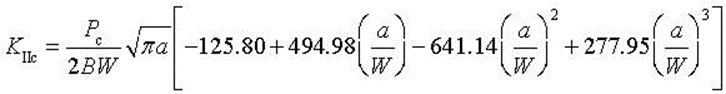(14)

Figure 9 shows the KIIca/W relationships obtained by the AFPB and 3ENF tests. When applying Eq. (14) to the results of the AFPB test, the values of KIIc obtained using the specimen with a depth value, W, of 40 mm are significantly larger than those obtained by specimens with a W value of 20 mm. This tendency is common for both results obtained from the groove-free and side-grooved specimens. Interestingly, the dependence of KIIc on a/W is not significant in the results obtained using the groove-free specimens, whereas the KIIc value decreases as the a/W increases in the results obtained from the side-grooved specimens. The KIIc value obtained from the groove-free specimen is larger than that obtained from the side-grooved specimen for the same a/W value. As described earlier, a density profile usually exists in MDF about its thickness, so the region with a higher density is removed when cutting a groove on the side surface of MDF (Bodig and Jayne 1982; Schulte and Früwald 1996; Dunky and Niemz 2002). The KIIc value obtained from the side-grooved specimen is, therefore, smaller than that of the groove-free specimen.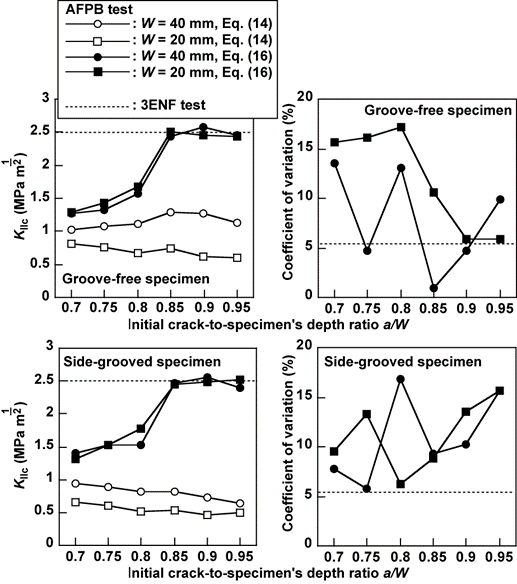Fig. 9. The comparison of the KIIca/W relationships obtained by the asymmetric four-point bending (AFPB) test of a single-edge-notched (SEN) specimen and a three-point bend end-notched flexure (3ENF) test. The additional crack lengths, each defined as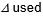in Eq. (16) were found to be 3.7 and 3.4 mm for specimens with depths of 40 and 20 mm, respectively.

When comparing the KIIc values obtained from the AFPB tests using the groove-free and side-grooved specimens and the 3ENF tests using the side-grooved specimens, the KIIc value obtained from the AFPB tests was definitively smaller than that obtained from the 3ENF tests. According to previous studies on the Mode I tests of solid wood and MDF and the Mode II test of solid wood, a cracked specimen often behaves as if the crack were longer than the actual length because of deformation around the crack tip, deflection caused by the shearing force, and development of a fracture process zone (FPZ) at the crack tip (Irwin 1961; Bažant and Kazemi 1990; Bao and Suo 1992; Ehart et al.1996; Stanzl-Tschegg et al. 1996; Ekberg 1997; Daudeville 1999; Bažant 2002; Vasic and Smith 2002, 2003; Vasic et al. 2002; Anderson 2005; de Moura et al. 2006, 2008, 2010; Silva et al. 2006; Yoshihara and Satoh 2009; Dourado et al. 2010; Susanti et al. 2010, 2011; Yoshihara 2010, 2012; Nakao et al. 2012). In these studies, the crack propagated straight from the crack tip. In contrast, Bažant and Pfeiffer (1986) suggest that the concept of a FPZ is also applicable for the asymmetric four-point loading of concrete where the kink crack is produced; therefore, it may be feasible that the cracked AFPB specimens of MDF also behave as if the crack were longer than the actual length. Further research must be conducted to determine whether this concept is applicable for the loading condition where the kink crack is produced. Similar to the approach adopted in previous studies (Yoshihara 2010, 2012; Yoshihara and Usuki 2011; Susanti et al. 2010, 2011; Nakao et al. 2012), the relationship between KIIc and a is represented by introducing an additional crack length, into Eq. (1), as follows: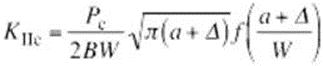(15)

Therefore, Eq. (14) is modified as follows: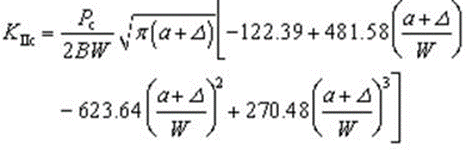(16)

The appropriate value of the additional crack length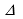is determined by the following procedure, which is similar to that adopted in previous works (Yoshihara 2010b, c; 2012; Yoshihara and Usuki 2011): (1) for various values of, calculate the probability value (p-value) for the average values of KIIc obtained by the AFPB test corresponding to each a/W and 3ENF test by conducting Student’s t-test and (2) sum the p-values corresponding to each. For large p-values, the average values of KIIcobtained by the AFPB and the 3ENF tests can be regarded as close to one another. With this procedure, it was determined that the additional crack lengths,are 3.7 and 3.4 mm for the groove-free specimens with the larger and smaller sizes, respectively, and 6.0 and 4.1 mm for the side-grooved specimen with the larger and smaller sizes, respectively. Figure 8 also shows the values of KIIccalculated using Eq. (16); these values are close to those obtained from the 3ENF tests. A statistical analysis of the difference between the KIIc values obtained using the AFPB and the 3ENF tests shows that the difference is not significant in the a/W range from 0.85 to 0.95. When the crack length is short, however, the load for inducing material nonlinearity may be smaller than that for inducing the crack propagation; therefore, when a/W is smaller than 0.8, the onset of nonlinearity is not due to propagation of the crack but rather due to the initiation of material nonlinearity.

Table 2 shows the Mode I and Mode II fracture toughness and critical stress intensity factor values obtained in a previous study (Yoshihara 2010a) and in the present study. Under pure Mode I conditions, KII = 0 and h1) = 1 because the crack propagates straight and forward ahead of the crack tip (= 0), so Eq. (2) is simplified as follows:(17)

Similar to solid wood, the GIIc value of MDF is significantly larger than the GIc value. Because of the difference between the crack paths under the Mode I and Mode II conditions, however, the Mode I and Mode II critical stress intensity factors are derived by Eqs. (17) and (5), respectively. As shown in Table 2, the difference between the KIc and KIIc values tends to be smaller than that between the GIcand GIIc values.

Table 2. The Mode I and Mode II Fracture Toughness and Critical Stress Intensity Factors of MDF Obtained in the Present Study and a Previous Study (Yoshihara 2010a)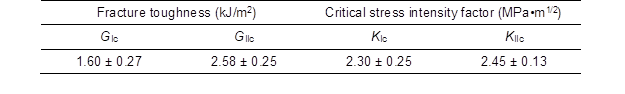* Mode I Fracture Toughness: GIc; Mode II Fracture Toughness: GIIc

* Mode I Critical Stress Intensity Factor: KIc; Mode II Critical Stress Intensity Factor: KIIc

In the AFPB test results of spruce obtained in the previous study, in which the crack propagated straight and forward from the crack tip, the values of additional crack lengths, each defined as, were 3.4 and 1.9 mm for specimens with depths of 40 and 20 mm, respectively (Yoshihara 2012). As described previously, however, the dependence of thevalue on the specimen’s dimensions is not as significant in MDF specimens. There are several reasons for the insignificant dependence such as the material in-plane isotropy, the kink crack formation, and the characteristics of MDF itself, such as its density profile (Bodig and Jayne 1982; Schulte and Früwald 1996; Dunky and Niemz 2002). As described earlier, it is still uncertain whether this correction is applicable for the loading condition in which the kink crack is produced, although the additional crack length is provisionally introduced in this research. Additionally, thevalue obtained in the 3ENF test, a large part of which might be because of the size of FPZ, was about 90 to 100 mm (Yoshihara 2010a), so it was extremely longer than thevalue obtained in this study.

From the results obtained in this study, it is difficult to reveal the reason for the difference ofvalues obtained from the 3ENF and AFPB tests, and the difficulty is more enhanced by the kink crack propagation. Microscopic observation (Niemz et al. 1999; Vasic and Smith 2002, 2003; Vasic et al.2002), full-field deformation observation techniques, such as digital image correlation (DIC) and electronic speckle pattern interferometry (ESPI) (Nairn 2009; Matsumoto and Nairn 2009), and FEA considering the FPZ (Daudeville 1999; de Moura et al. 2006, 2008, 2010; Silva et al. 2006; Dourado et al 2010) may reveal the influence of the microstructure and the size of the FPZ on the value of, as well as the validity of the concept of additional crack length itself.

CONCLUSIONS

Using single-edge-notched (SEN) specimens composed of medium-density fiberboard (MDF), asymmetric four-point bending (AFPB) tests were conducted to measure the Mode II critical stress intensity factor. The conclusions are summarized as follows:

1. In the AFPB loading of the MDF specimens, a kink crack was always produced. Kink crack formation should therefore be taken into account in the analysis of the Mode II fracture mechanics in AFPB tests.

2. The FEA results indicated that the Mode I strain energy release rate is dominant after the propagation of the kink crack; therefore, it is difficult to obtain the R-curve of MDF in a pure Mode II condition, despite the fact that the crack initially propagated under a pure Mode II condition.

3. The results of the Mode I fracture tests (SENB, SENT, and CT tests) conducted in previous studies indicated that the critical stress intensity factor KIc can be obtained effectively by introducing an additional crack length,. This concept was also effective for the measurement of the Mode II critical stress intensity factor KIIc in spite of the kink crack formation, although further research should be conducted to reveal the validity of this concept.

ACKNOWLEDGEMENT

A part of this work was supported by Grant-in-Aid for Scientific Research (C) (No. 21580207) of the Japan Society for the Promotion of Science (JSPS).

References cited

Adams, D. F., Carlsson, L. A., and Pipes, R. B. (2003). Experimental Characterization of Advanced Composite Materials 3rd Ed., CRC Press, Boca Raton.

Anderson, T. L. (2005). Fracture Mechanics: Fundamentals and Applications 3rd Ed., Taylor & Francis, Boca Raton.

Bao, G., and Suo, Z. (1992). “Remarks on crack-bridging concepts,” Appl. Mech. Rev. 45, 355-366.

Bažant, Z. P. (2002). “Concrete fracture model: Testing and practice,” Eng. Fract. Mech. 69, 165-205.

Bažant, Z. P., and Kazemi, M. T. (1990). “Size effect in fracture of ceramics and its use to determine fracture energy and effective process zone length,” J. Am. Ceram. Soc. 73, 1841-1853.

Bažant, Z. P., and Pfeiffer, P. A. (1986). “Shear structure tests of concrete,” Mater. Struct. 19, 111-121.

Bilby, B. A., and Cardew, G. E. (1975). “The crack with a kinked tip,” Int. J. Fract. 11, 708-711.

Bodig, J., and Jayne, B. A. (1982). Mechanics of Wood and Wood Composites, Van Nostrand, New York.

Choi, S. R., Zhu, D., and Miller, R. A. (2003). “Mode I, mode II, and mixed-mode fracture of plasma-sprayed thermal barrier coatings at ambient and elevated temperatures,” NASA TM-2003-212185

Choi, S. R., Zhu, D., and Miller, R. A. (2005). “Fracture behavior under mixed-mode loading of ceramic plasma-sprayed thermal barrier coatings at ambient and elevated temperatures,” Eng. Fract. Mech. 72, 2144-2158.

Daudeville, L. (1999). “Fracture in spruce: Experiment and numerical analysis by linear and non linear fracture mechanics,” Holz Roh- Werkst. 57, 425-432.

Davies, P, Blackman, B. R. K., and Brunner, A. J. (2001). “Mode II delamination,” In: Moore, D. R., Pavan, A., Williams, J. G., (ed.), Fracture Mechanics Testing Methods for Polymers Adhesive and Composites ESIS Publication 28. Elsevier, Amsterdam.

de Moura, M. F. S. F., Silva, M. A. L., de Morais A. B., and Morais, J. J. L. (2006). “Equivalent crack based mode II fracture characterization of wood,” Eng. Fract. Mech. 73, 978-993.

de Moura , M. F. S. F., Morais, J. J. L., and Dourado, N. (2008). “A new data reduction scheme for mode I wood fracture characterization using the double cantilever beam test,” Eng. Fract. Mech. 75, 3852-3865.

de Moura, M. F. S. F., Dourado, N., and Morais, J. J. L. (2010). “Crack equivalent based method applied to wood fracture characterization using the single edge notched-three point bending test,” Eng. Fract. Mech. 75, 510-520.

Dourado, N., de Moura, M. F. S. F., Morais, J. J. L., and Silva, M. A. L. (2010). “Estimate of resistance-curve in wood through the double cantilever beam test,” Holzforschung 64, 119-126.

Dunky, M., and Niemz, P. (2002). Holzwerkstoffe und Leime, Springer, Berlin.

Ehart, R. J., Stanzl-Tschegg, S. E., and Tschegg, E. K. (1996). “Characterization of crack propagation in particleboard,” Wood Sci. Technol. 30, 307-321.

Ekberg, A. (1997). Fracture Mechanics – Some notes-, http://www.am.chalmers.se/~anek/research/fm.pdf

Fett, T., Gerteisen, G. Hahnenberger, S., Martin, G., and Munz, D. (1995). “Fracture tests for ceramics under mode-I, mode-II and mixed-mode loading,” J. Eur. Ceram. Soc. 15, 307-312.

Hayashi, K., and Nemat-Nasser, S. (1981). “Energy-release rate and crack kinking under combined loading,” Trans. ASME J. Appl. Mech. 48, 520-524.

He, M. Y., and Hutchinson, J. W. (2000). “Asymmetric four-point crack specimen,” Trans. ASME J. Appl. Mech. 67, 207-209

Irwin, G. R. (1961). “Plastic zone near a crack and fracture toughness,” Sagamore Res. Confer. Proc.4, 63-78.

Kageyama, K., and Okamura, H. (1982). “Elastic analysis of infinitesimally kinked crack under tension and transverse shear and the maximum energy release rate criterion,” Trans. Jpn. Soc. Mech. Eng. A 4, 783-791.

Kazemi Najafi, S., Abbasi Marasht, A., and Ebrahimi, G. (2007). “Prediction of ultrasonic wave velocity in particleboard and fiberboard,” J. Mater. Sci. 42, 789-793.

Kitahara, K. (1963). “On the heterogeneity of fiberboard and particleboard,” J. Soc. Mater. Sci. Jpn. 12, 722-726.

Krishnan, A., and Xu, L. R. (2010). “A short-beam shear fracture approach to measure the mode II fracture toughness of materials with preferred interfaces,” Int. J. Fract. 169, 15-25.

Li, M., and Sakai, M. (1996). “Mixed-mode fracture of ceramics in asymmetric four-point bending: Effect of crack-face grain interlocking/bridging,” J. Am. Ceram. Soc. 79, 2718-2726.

Matsumoto, N., and Nairn, J. A. (2009). “The fracture toughness of medium density fiberboard (MDF) including the effects of fiber bridging and crack-plane interference,” Eng. Fract. Mech. 76, 2748-2757.

Nairn, J. A. (2009). “Analytical and numerical modeling of R curves for cracks with bridging zones,” Int. J. Fract. 155, 167-181.

Niemz, P., Diener, M., and Pöhler, E. (1997). “Untersuchungen zur Ermittlung der Bruchzähigkeit an MDF-Platten,” Holz Roh Werkst. 55, 327-330.

Niemz, P., Diener, M., and Pöhler, E. (1999). “Vergleichende Untersuchungen zur Ermittlung der Bruchzähigkeit an Holzwerkstoffen,” Holz Roh Werkst. 57, 222-224

Nakao, T., Susanti, C. M. E., and Yoshihara, H. (2012). “Examination of the failure behavior of wood with a short crack in the radial-longitudinal system by single-edge-notched bending test,” J. Wood Sci. 58, 453-458.

Nuismer, R. J. (1974). “An energy release rate criterion for mixed mode fracture,” Int. J. Fract. 10, 305-321.

Olbert, B. H., and Schenck, S. R. (1997). “Fracture of an aluminosilicate fiberboard,” J. Am. Ceram. Soc. 80, 2789-2797.

Olbert, B. H. (1999). “Transverse fracture of an aluminosilicate fiberboard,” J. Am. Ceram. Soc. 82, 414-420.

Rybicki, E. F., and Kanninen, M. F. (1977). “A finite element calculation of stress intensity factors by a modified crack closure integral,” Eng. Fract. Mech. 9, 931-938.

Schulte, M., and Früwald, A. (1996). “Shear modulus, internal bond and density profile of medium density fibre board (MDF),” Holz Roh- Werkst. 54, 49-55.

Silva, M. A. L., de Moura, M. F. S. F., and Morais, J. J. L. (2006). “Numerical analysis of the ENF test for mode II wood fracture,” Composites A 37, 1334-1344.

Stanzl-Tschegg, S. E., Tan, D. M., and Tschegg, E. K. (1996). “Fracture resistance to the crack propagation in wood,” Int. J. Fract. 75, 347-356.

Suresh, S., Shih, C. F., Morrone, A., and O’Dowd, N. P. (1990). “Mixed-mode fracture toughness of ceramic materials,” J. Am. Ceram. Soc. 73, 1257-1267.

Susanti, C. M. E., Nakao, T., and Yoshihara, H. (2010). “Examination of the failure behaviour of wood with a short crack in the tangential-radial system by single-edge-notched bending test,” Eng. Fract. Mech. 77, 2527-2536.

Susanti, C. M. E., Nakao, T., and Yoshihara, H. (2011). “Examination of the Mode II fracture behaviour of wood with a short crack in an asymmetric four-point bending test,” Eng. Fract. Mech. 78, 2775-2788.

Suzuki, N., and Matsushita, Y. (1998). “Fracture toughness of wood-based composite boards in opening mode I measurement of fracture toughness of various wood-based composite boards,” Bull. Mie Univ. Forests 22, 101-106.

Vasic, S., and Smith, I. (2002). “Bridging crack model for fracture of spruce,” Eng. Fract. Mech. 69, 745-760.

Vasic, S., and Smith, I. (2003). “Contact – crack problem with friction in spruce,” Holz Roh- Werkst 61, 182-186.

Vasic, S., Smith, I., and Landis, E. (2002). “Fracture zone characterization – micro-mechanical study,”Wood Fiber Sci 34, 42-56.

Xie, D., Waas, A. M., Shahwan, K. W., Schroeder, J. A., and Boeman, R. G. (2004). “Computation of energy release rates for kinking cracks based on virtual crack closure technique,” Comput. Model. Eng. Sci. 6, 515-524.

Yoshihara, H. (2008). “Mode II fracture mechanics properties of wood measured by asymmetric four-point bending test using a single-edge-notched specimen,” Eng. Fract. Mech. 75, 4727-4739.

Yoshihara, H. (2010a). “Mode I and mode II initiation fracture toughness and resistance curve of medium density fiberboard measured by double cantilever beam and three-point bend end-notched flexure tests,” Eng. Fract. Mech. 77, 2537-2549.

Yoshihara, H. (2010b). “Examination of the mode I critical stress intensity factor of wood obtained by single-edge-notched bending test,” Holzforschung 64, 501-509.

Yoshihara, H. (2010c). “Influence of loading conditions on the measurement of mode I critical stress intensity factor for wood and medium-density fiberboard by the single-edge-notched tension test,” Holzforschung 64, 735-745.

Yoshihara, H. (2012). “Mode II critical stress intensity factor of wood measured by the asymmetric four-point bending test of single-edge-notched specimen while considering an additional crack length,” Holzforschung 66, 989-992.

Yoshihara, H., and Satoh, A. (2009). “Shear and crack tip deformation correction for the double cantilever beam and three-point end-notched flexure specimens for mode I and mode II fracture toughness measurement of wood,” Eng. Fract. Mech. 76, 335-346.

Yoshihara, H., and Usuki, A. (2011). “Mode I critical stress intensity factor of wood and medium-density fiberboard measured by compact tension test,” Holzforschung 65, 729-735.

Article submitted: November 28, 2012; Peer review completed: February 9, 2013; Revised version received: February 14, 2013; Accepted: February 15, 2013; Published: February 19, 2013.